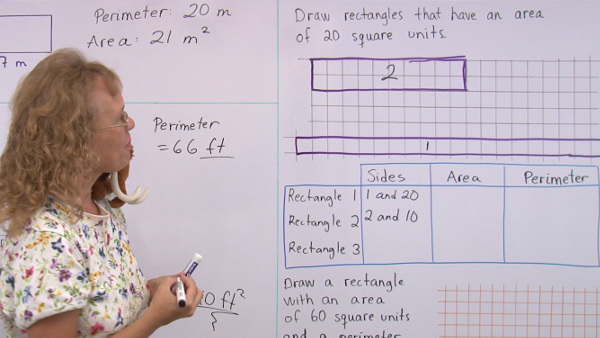THANKSGIVING SALE! Get Math Mammoth and Make It Real Learning downloads & CDs at 25% off — right here at the MathMammoth.com website. Use coupon code DVTF-KCW4-M3WX at checkout. Offer valid through December 1, 2020.
^

# Video lessons for elementary geometry topicsThis is a collection of free video lessons for elementary and middle school geometry topics (grades 1-7). Please choose a topic from the list below.

## Area and perimeter: grades 3-5

Perimeter

Units of area

Problems to solve: area of rectangles

Area of rectangles and the distributive property

Area and perimeter problems — rectangular shapes

Area versus perimeter

Lines, rays, and angles

How to use a protractor to measure & draw angles

Estimating angles

How to draw a right angle (perpendicular line) and a rectangle

Parallelograms

Acute, obtuse, and right triangles

Polygons—introduction

Circles

Area and perimeter problems

Classify triangles

Volume of a rectangular prism and cubic units

Area of parallelograms and triangles

Area of polygons

Find the area of a triangle by drawing and measuring the altitude

Introduction to conversions between units of area

Volume of rectangular prism with fractional edge length

Unknown angle problems

Angles in a triangle

Drawing geometric shapes with given conditions

Drawing triangles with given conditions

Pi and the circumference of a circle

How to calculate the area of a circle, plus simple proof for the formula

The Pythagorean Theorem

Applications of the Pythagorean Theorem 1: area of an isosceles triangle

Applications of the Pythagorean Theorem 2: diagonal of a square

Applications of the Pythagorean Theorem 3: volume of a triangular prism

Applications of the Pythagorean Theorem 4: perimeter of a trapezoid

Back to the videos index

WAIT!

Receive my monthly collection of math tips & resources directly in your inbox — and get a FREE Math Mammoth book!You can unsubscribe at any time.

### Math Mammoth Tour

Confused about the different options? Take a virtual email tour around Math Mammoth! You'll receive:

An initial email to download your GIFT of over 400 free worksheets and sample pages from my books. Six other "TOURSTOP" emails that explain the important things and commonly asked questions concerning Math Mammoth curriculum. (Find out the differences between all these different-colored series!)

This way, you'll have time to digest the information over one or two weeks, plus an opportunity to ask me personally about the curriculum.
A monthly collection of math teaching tips & Math Mammoth updates (unsubscribe any time)### "Mini" Math Teaching Course

This is a little "virtual" 2-week course, where you will receive emails on important topics on teaching math, including:

- How to help a student who is behind
- Troubles with word problems
- Teaching multiplication tables
- Why fractions are so difficult
- The value of mistakes
- Should you use timed tests
- And more!

A GIFT of over 400 free worksheets and sample pages from my books right in the very beginning.A monthly collection of math teaching tips & Math Mammoth updates (unsubscribe any time)
Enter your email to receive math teaching tips, resources, Math Mammoth news & sales, humor, and more! I tend to send out these tips about once monthly, near the beginning of the month, but occasionally you may hear from me twice per month (and sometimes less often).• A GIFT of over 400 free worksheets and sample pages from my books.